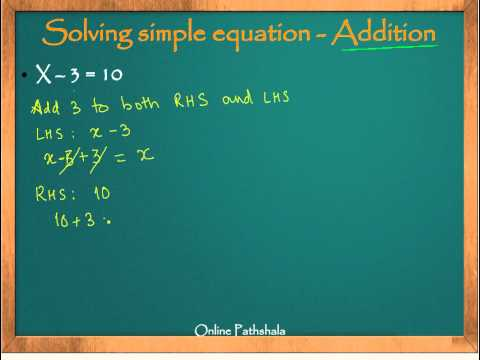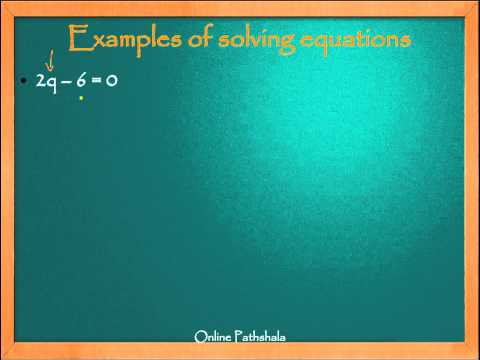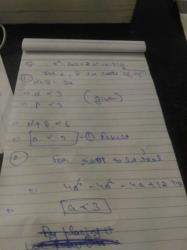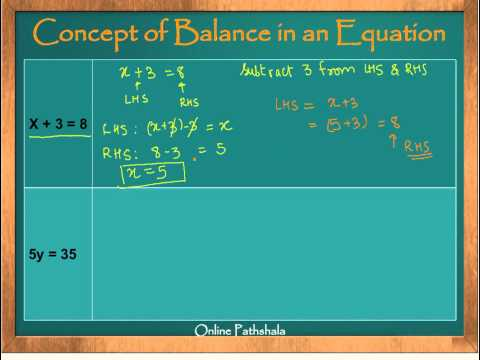Courses

# Test: Simple Equations

## 15 Questions MCQ Test NCERT Mathematics for CAT Preparation | Test: Simple Equations

Description
This mock test of Test: Simple Equations for Class 7 helps you for every Class 7 entrance exam. This contains 15 Multiple Choice Questions for Class 7 Test: Simple Equations (mcq) to study with solutions a complete question bank. The solved questions answers in this Test: Simple Equations quiz give you a good mix of easy questions and tough questions. Class 7 students definitely take this Test: Simple Equations exercise for a better result in the exam. You can find other Test: Simple Equations extra questions, long questions & short questions for Class 7 on EduRev as well by searching above.
QUESTION: 1

### Write the statements “The sum of three times x and 11 is 32” in the form of equations:

Solution:

The sum of three times x and 11 is 32.
⇒ 3x + 11 = 32

QUESTION: 2

### Write the statements “If you subtract 5 from 6 times a number, you get 7” in the form of equations:

Solution:

Let us say the number is x;
⇒ x multiplied by 6 is 6x.
On Subtracting 5 from 6x, one gets 6x – 5.
∵ The result is 7.
∴ The equation is 6x – 5 = 7

QUESTION: 3

### Write the statements “One fourth of m is 3 more than 7” in the form of equations:

Solution:

Let's approach the statement stepwise:
► One-fourth of m = m/4
► 3 more than 7 will be 3 + 7

Equating both sides, m/4 = 3 + 7

QUESTION: 4

Write the statements “Product of One third of a number and m plus 5 is 8” in the form of equations:

Solution:

One-third of a number plus 5 is 8
⇒ (1/3) m + 5 = 8

QUESTION: 5

Which is a solution of the equation 2x = 12?

Solution:

Given that, 2x = 12
⇒  x = 6

QUESTION: 6

Which is a solution of the equation x + 4 = 6?

Solution:

Given that,  x + 4 = 6
⇒ x = 6 - 4 = 2

QUESTION: 7

Which is a solution of the equation 7x + 5 = 19?

Solution:

Given that, 7x + 5 = 19
⇒ 7x = 19 - 5 = 14
⇒  x = 2

QUESTION: 8

Which is a solution of the equation 4x – 3 = 13?

Solution:

Given that, 4x – 3 = 13
⇒ 4x = 13 + 3  = 16
⇒ x = 4

QUESTION: 9

Which is a solution of the equation 5x + 2 = 17?

Solution:

Given that, 5x + 2 = 17
⇒ 5x = 17 - 2 = 15
⇒ x = 3

QUESTION: 10

Which is a solution of the equation 3x – 14 = 4?

Solution:

Given that, 3x - 14 = 4
⇒ 3x = 18
⇒ x = 6

QUESTION: 11

One - fifth of a number minus 4 gives 3. Find which of the following is the number?

Solution:

Let the number be x.
Equation: (1/5) x - 4 = 3
⇒ (1/5) x = 4 + 3  = 7
⇒ x = 35

QUESTION: 12

Which of the following is not allowed in any equation?
(a) Adding the same number to both sides of the equation.
(b) Subtracting the same number from both sides of the equation.
(c) Multiplying both sides of the equation by the same non-zero number.
(d) Dividing both sides of the equation by the same number.

Solution:

Division of any number by zero is not allowed, therefore dividing both sides of the equation by the same non-zero number is allowed in a given equation.

QUESTION: 13

Subtracting 5 from p , the result is 3. What is the value of p?

Solution:

Given: p - 5 = 3
⇒ p = 5 + 3 = 8

QUESTION: 14

13 subtracted from twice a number gives 2. What is the number?

Solution:

Let the number be x.
Equation: 2x - 13 = 2
⇒ 2x = 2 + 13 = 15
⇒ x = 15/2

QUESTION: 15

5 times a number increased by 5 is 25. What is the number?

Solution:

Let the number be x
Equation: 5x + 5 = 25
⇒ 5x = 20
⇒ x = 4

Track your progress, build streaks, highlight & save important lessons and more!

### Similar Content### Related tests